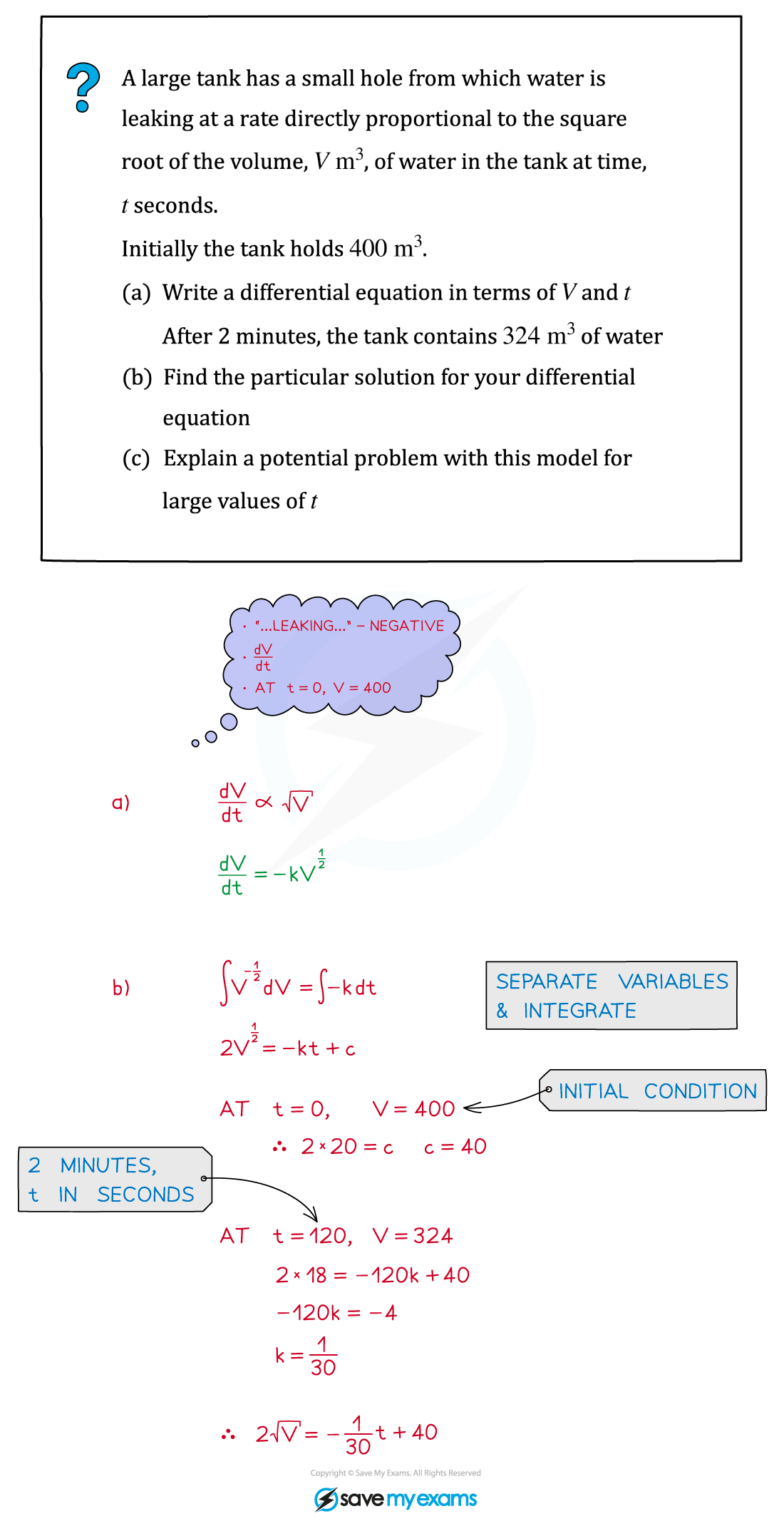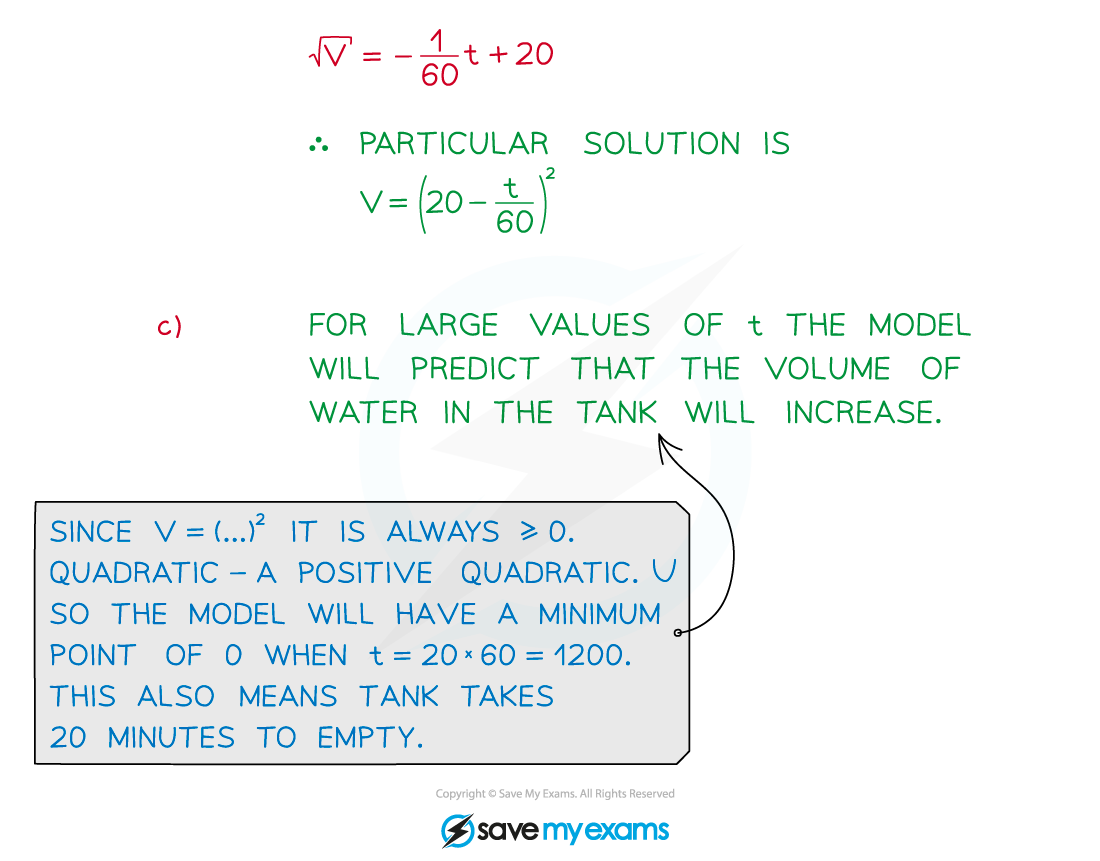# CIE A Level Maths: Pure 3复习笔记5.2.5 Solving & Interpreting Differential Equations

### Solving & Interpreting Differential Equations

#### How do I solve a differential equation?

• Solving differential equations uses integration!
• The precise integration method will depend on the type of question (see Decision Making)
• Separation of variables is highly likely to be involved
• Particular solutions are usually required to Differential Equations
• An initial/boundary condition is needed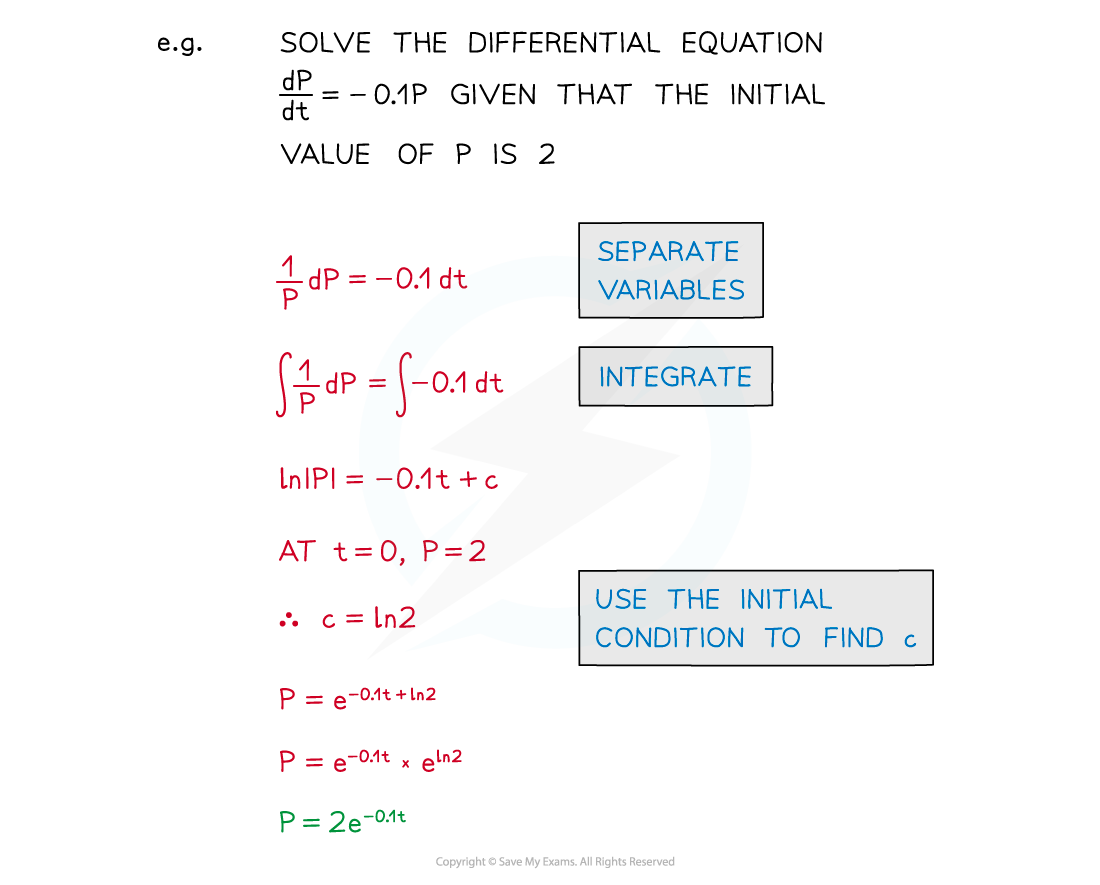• Solutions can be rewritten in a format relevant to the model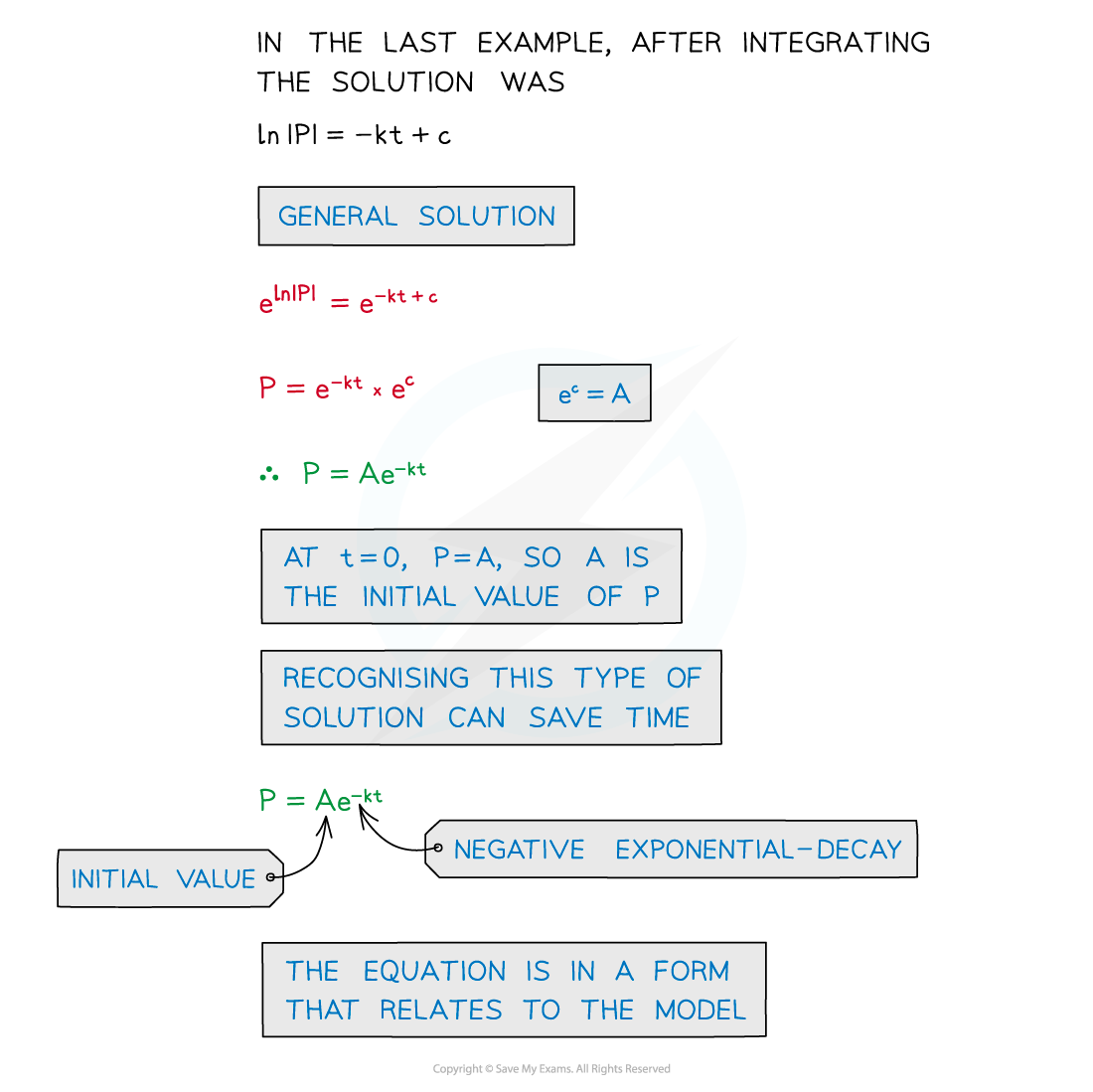• The solution can be used to make predictions at other times
• Temperature after four minutes
• Volume of sales after another three months

#### How do I use the solution to a differential equation?

• Questions may ask you to interpret your solutions in the context of the problem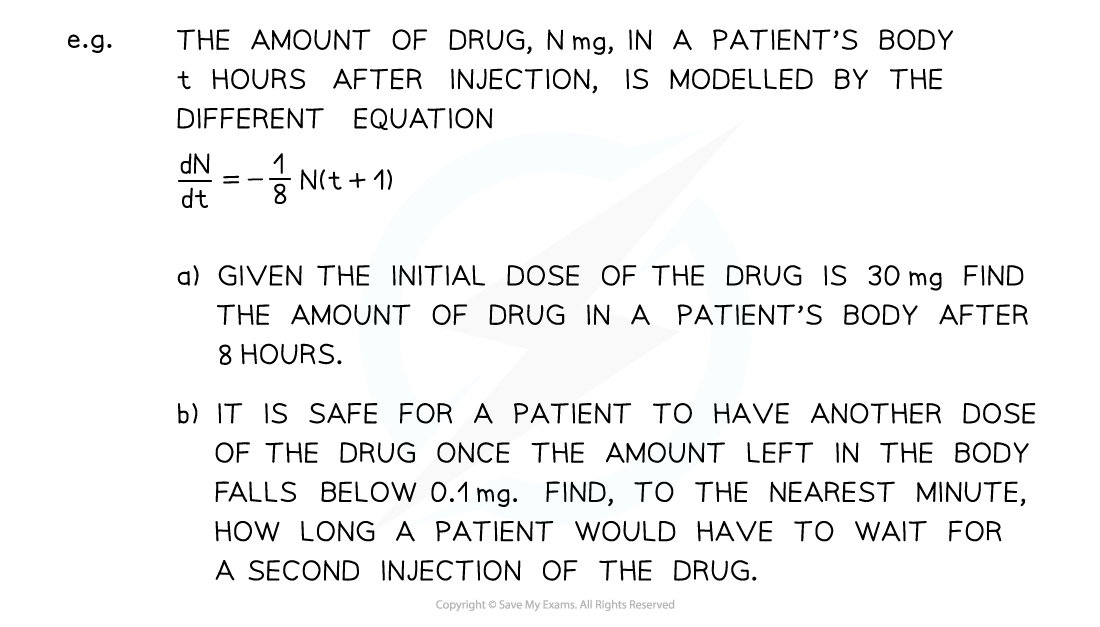• There could be links to other areas of A level maths – such as mechanics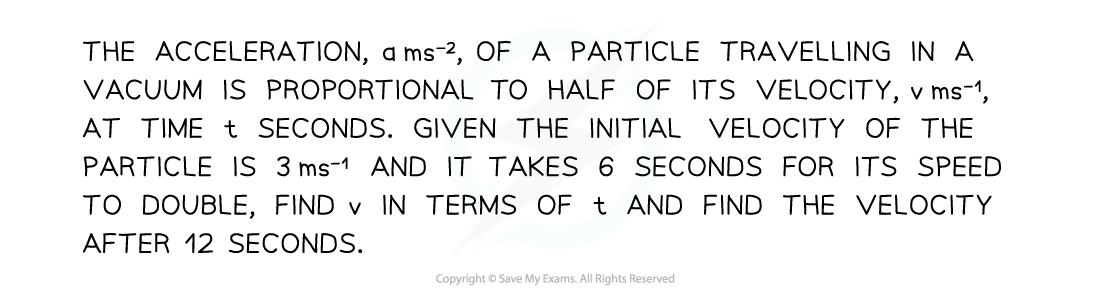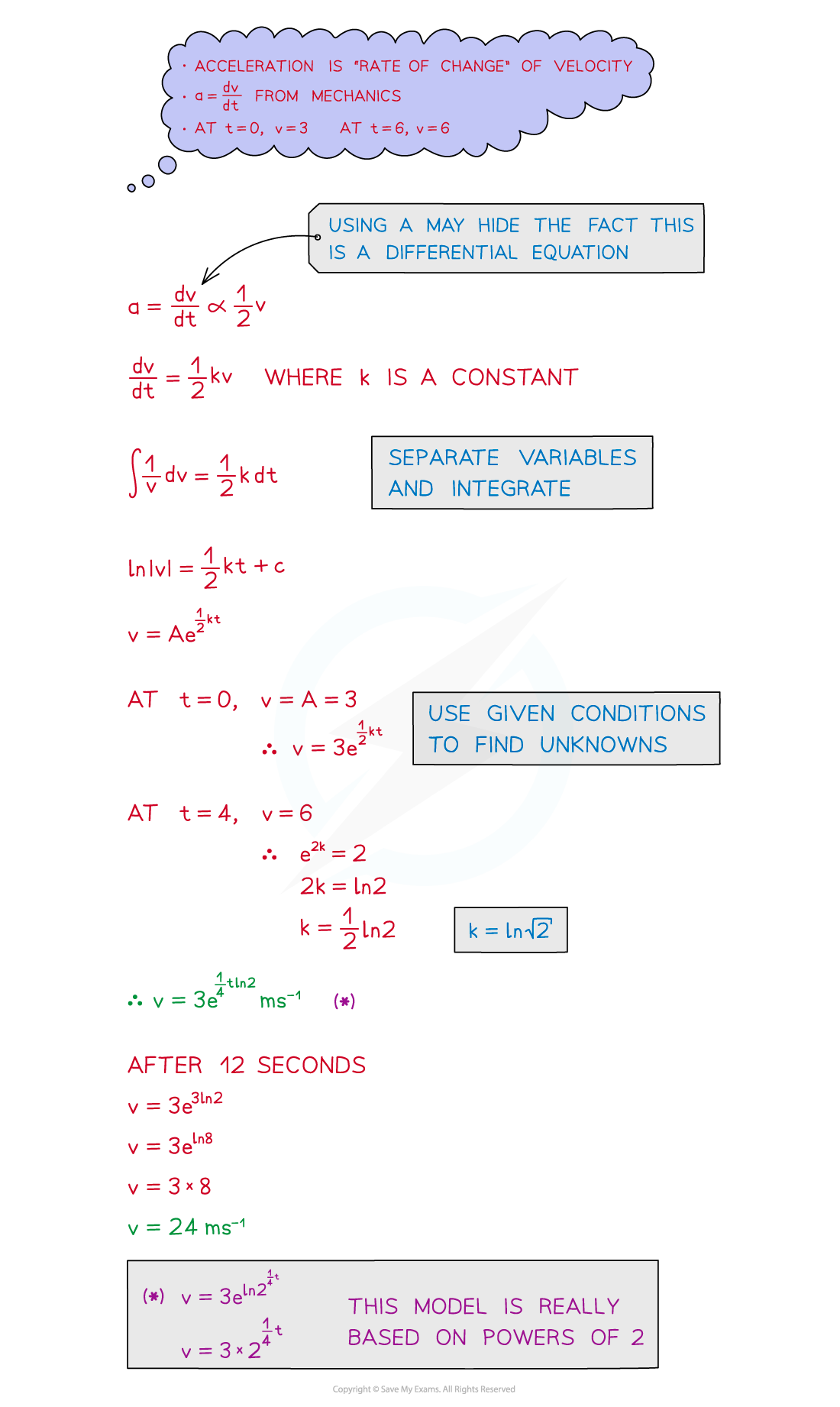• Sometimes multiple rates of change may be involved in a model or problem
• See Connected Rates of Change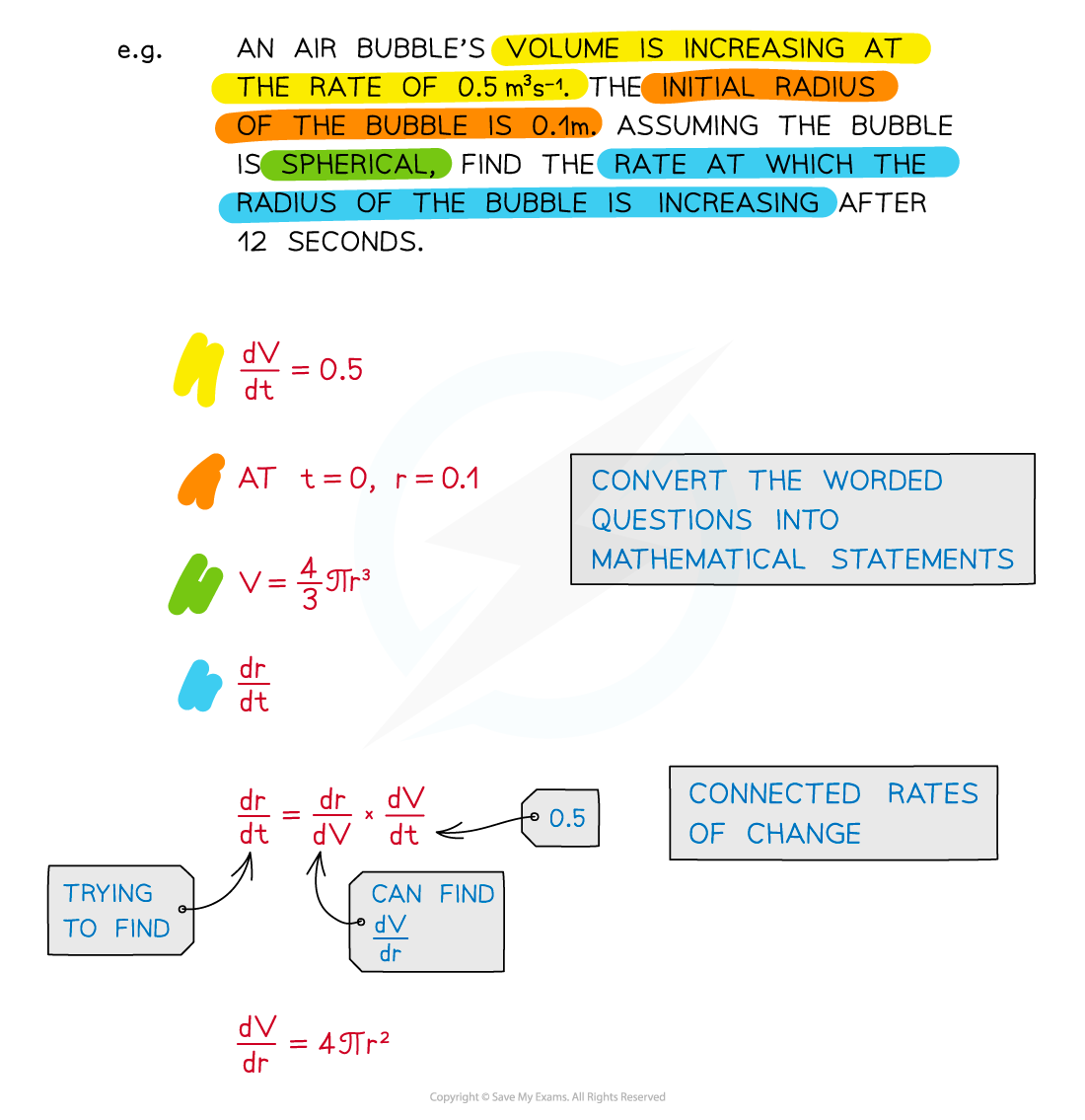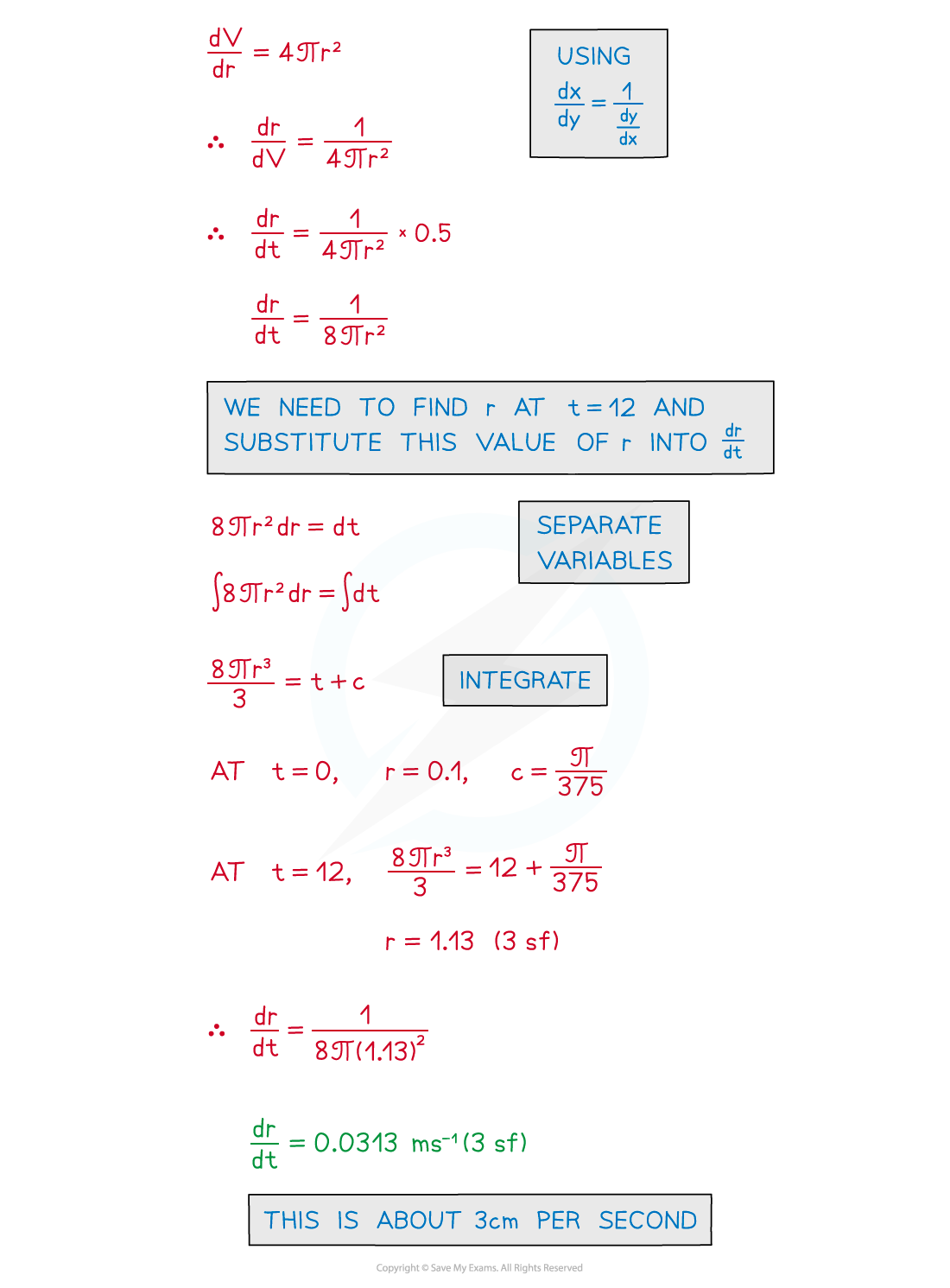#### How do I interpret a differential equation?

• Models may not always be realistic in the long term
• A population will not grow indefinitely – it will reach a natural limit
• You will be expected to interpret and comment on the model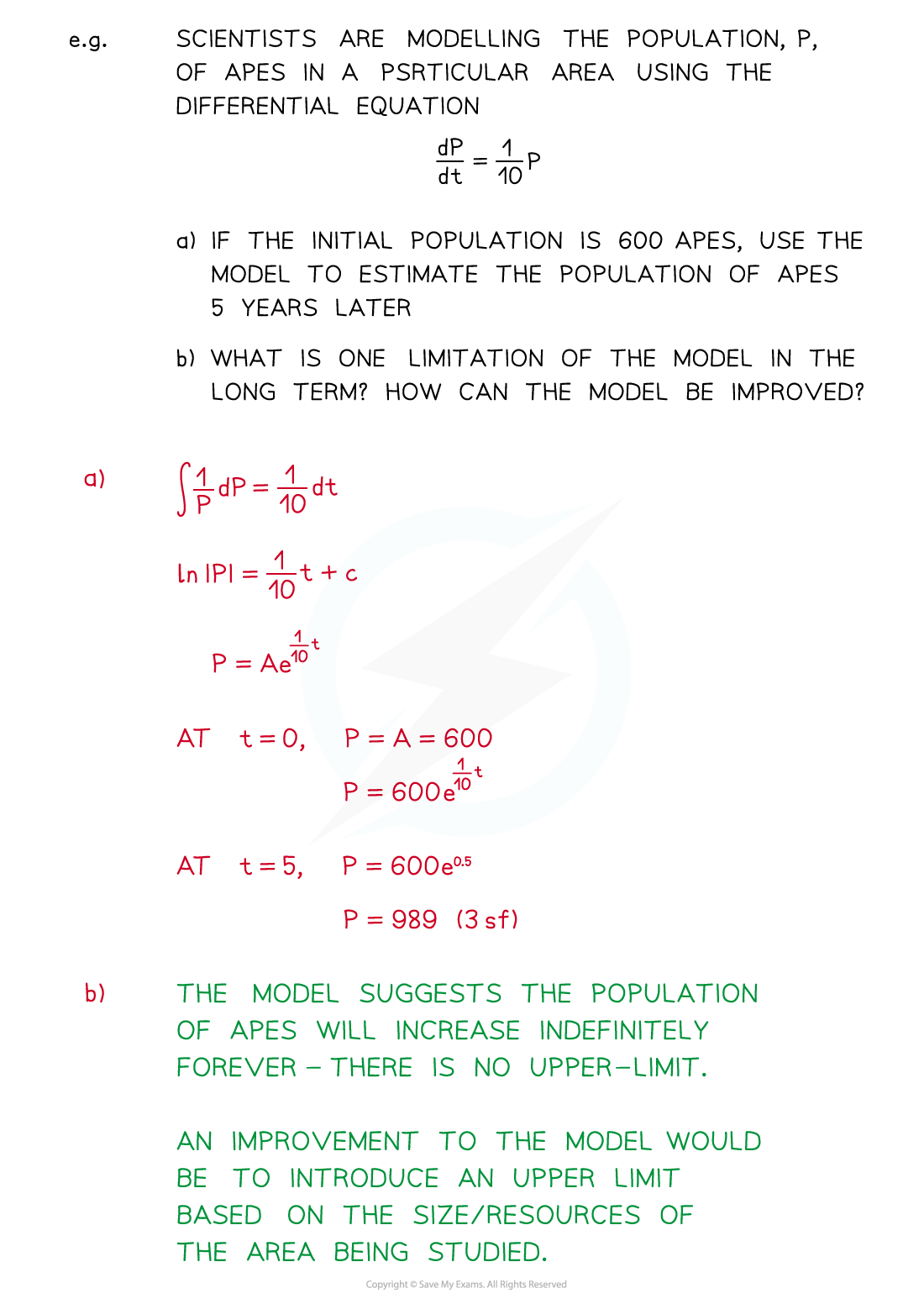#### Worked Example